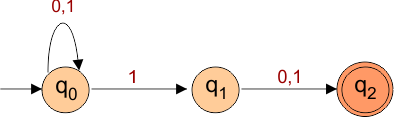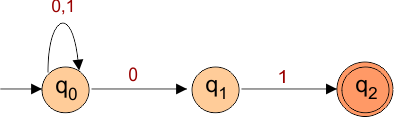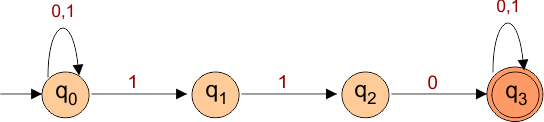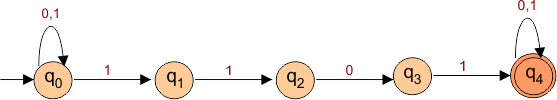Select Page

# Examples of NFA

As we already cover the topic of NFA in previous lecture. Let see some examples of Non deterministic Finite Automata (NFA).

## Example 01

Design a NFA of all binary strings in which 2nd last bit is 1.

### Solution

Language for given question is given below

L= {10, 010, 000010, 11, 101011……..}

## NFA for above language isExample 2

Design an NFA with ∑ = {0, 1} accepts all string ending with 01.

### Solution

Language for given question is given below

L= {01, 0101, 0000101, 101, 101001……..}

NFA for above language is## Example 3

Construct an NFA with ∑ = {0, 1} in which a substring “double ‘1’ is followed by single ‘0’” must exist.

### Solution

Language for given question is given below

L= {110, 0110, 1110 , 10100110……..}

NFA for above language is## Example 4

Construct an NFA in which all the string contains a substring 1101.

### Solution

Language for given question is given below

L= {1101, 110101, 1101000101, 01101, 1011011……..}

NFA for above language isHelp Other’s By Sharing…